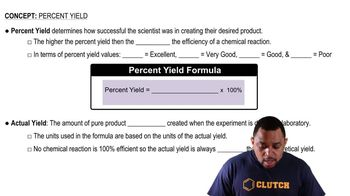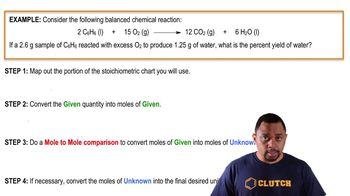Start typing, then use the up and down arrows to select an option from the list.
5:32 minutes
Problem 107a
Textbook Question

# Aspirin can be made in the laboratory by reacting acetic anhydride (C4H6O3) with salicylic acid (C7H6O3) to form aspirin (C9H8O4) and acetic acid (C2H4O2). The balanced equation is: C4H6O3 + C7H6O3¡C9H8O4 + C2H4O2 In a laboratory synthesis, a student begins with 3.00 mL of acetic anhydride (density = 1.08 g/mL) and 1.25 g of salicylic acid. Once the reaction is complete, the student collects 1.22 g of aspirin. Determine the limiting reactant. Determine the theoretical yield of aspirin. Determine the percent yield for the reaction.Verified Solution
This video solution was recommended by our tutors as helpful for the problem above.
1478views